# ETTORE SOTTSASS rare metal centerpiece Zani 2004 Artist’s Proof limited edition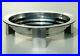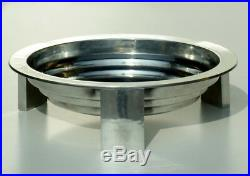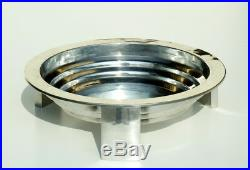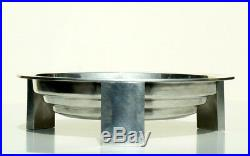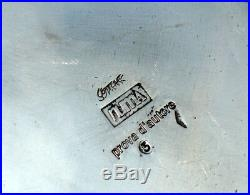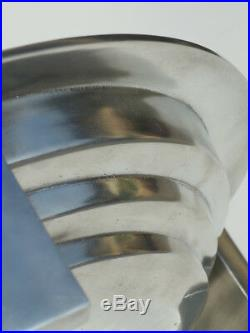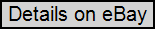” T A V O L A R O T O N D A R O V E S C I A “. E t t o r e S o t t s a s s. S e r a f i n o Z a n i – N U M A 2 0 0 4. C a s t p e w t e r h a n d – f i n i s h e d. W i t h b r u s h p o l i s h i n g. E x c e l l e n t C o n d i c t i o n. M E A S U R E S. D i a m 3 0 , 5 c m. W E S H I P I N W O R L D W I D E. S H I P. I N G C O S T. I T A L Y. E U R O P E. O T H E R. C O U N T R Y. P l e a s e. T E R M S O F S A L E. P A Y M E N T. P a y p a l – B a n k T r a n s f e r. P R O F E S S I O N A L P A C K E T. B u b b l e W r a p – P o l y s t y r e n e c u b e s. H a r d C a r d b o a r d B o x e s. C O M M U N I C A T I O N. P r o m p t a n s w e r w i t h i n 2 4 h o u r s. I t e m 4 8. The item “ETTORE SOTTSASS rare metal centerpiece Zani 2004 Artist’s Proof limited edition” is in sale since Wednesday, September 20, 2017. This item is in the category “Antiques\Periods & Styles\Mid-Century Modernism”. The seller is “tangram_xy07″ and is located in Milano. This item can be shipped worldwide.
• Type: centerpiece
• Sub-Style: Italian
• Original/Reproduction: Original
• ARTIST: Ettore Sottsass
• Material: Pewter polish
• Height: 8, 5 cm
• Maker: Serafino Zani _ Numa
• Diameter: 30, 5 cm